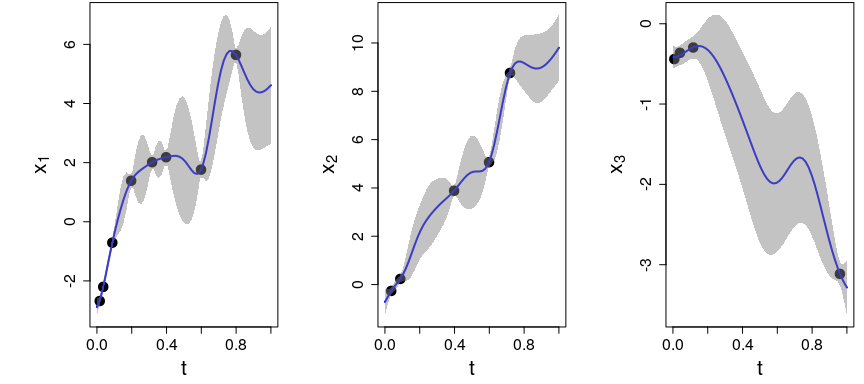# MGPR example

library(GPFDA)
require(MASS)

## Simulating data from a multiple GP with three outputs

In this example, we simulate data from a multivariate (convolved) GP model. See details of this model in Chapter 8 of Shi, J. Q., and Choi, T. (2011), “Gaussian Process Regression Analysis for Functional Data”, CRC Press.

We simulate $$30$$ realisations of three dependent outputs, with $$250$$ time points on $$[0,1]$$ for each output.

set.seed(123)
nrep <- 30
n1 <- 250
n2 <- 250
n3 <- 250
N <- 3
n <- n1+n2+n3
input1 <- sapply(1:n1, function(x) (x - min(1:n1))/max(1:n1 - min(1:n1)))
input2 <- input1
input3 <- input1

# storing input vectors in a list
Data <- list()
Data$input <- list(input1, input2, input3) # true hyperparameter values nu0s <- c(6, 4, 2) nu1s <- c(0.1, 0.05, 0.01) a0s <- c(500, 500, 500) a1s <- c(100, 100, 100) sigm <- 0.05 hp <- c(nu0s, log(nu1s), log(a0s), log(a1s), log(sigm)) # Calculate covariance matrix Psi <- mgpCovMat(Data=Data, hp=hp) We need an index vector identifying to which output the data corresponds: ns <- sapply(Data$input, length)
idx <- c(unlist(sapply(1:N, function(i) rep(i, ns[i])))) 

Covariance functions $$\text{Cov}\big[X_j(t), X_\ell(0) \big]$$ can be plotted as follows. The arguments output and outputp correspond to $$j$$ and $$\ell$$, respectively.

Given the hyperparameters hp, we can plot the auto- and cross-covariance functions as follows:

# Plotting an auto-covariance function
plotmgpCovFun(type="Cov", output=1, outputp=1, Data=Data, hp=hp, idx=idx)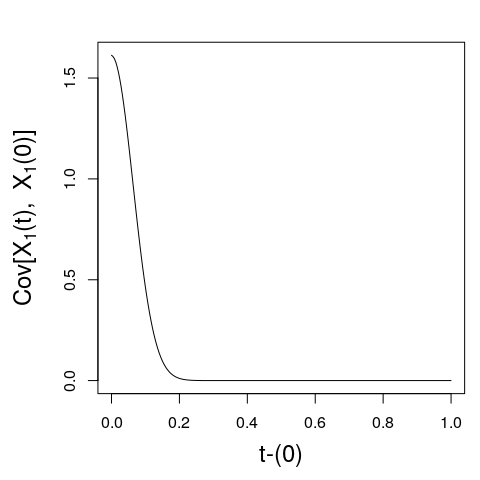# Plotting a cross-covariance function
plotmgpCovFun(type="Cov", output=1, outputp=2, Data=Data, hp=hp, idx=idx)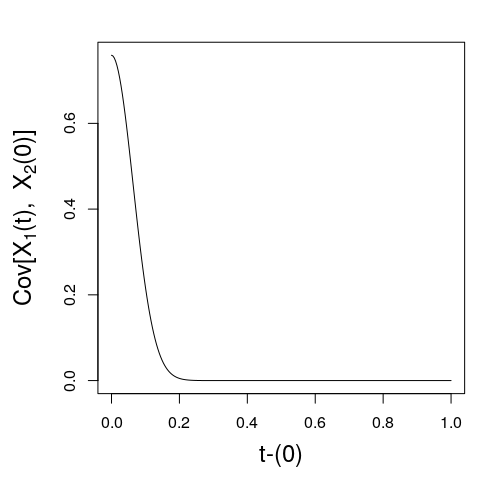Corresponding correlation functions can be plotted by setting type=Cor:

# Plotting an auto-correlation function
plotmgpCovFun(type="Cor", output=1, outputp=1, Data=Data, hp=hp, idx=idx)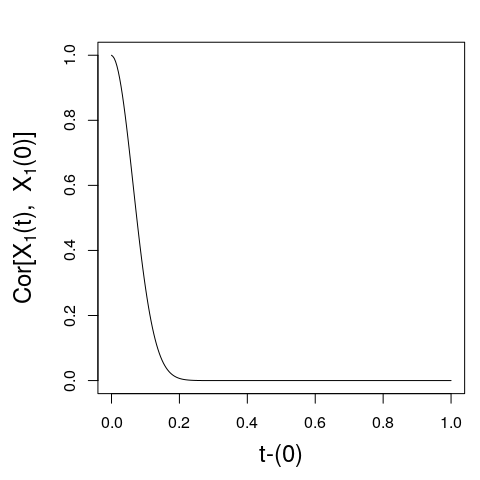# Plotting a cross-correlation function
plotmgpCovFun(type="Cor", output=1, outputp=2, Data=Data, hp=hp, idx=idx)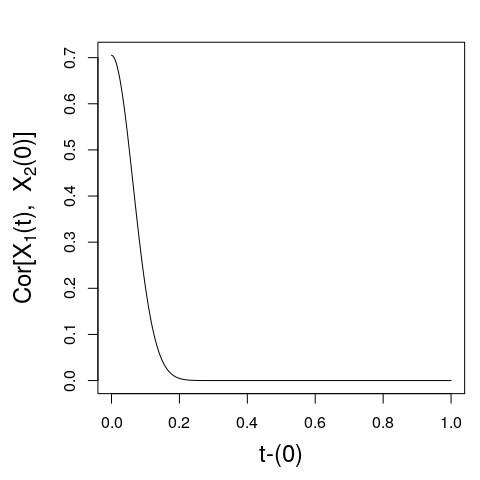We assume that the mean functions for each output are $$\mu_1(t) = 5t$$, $$\mu_2(t) = 10t$$, and $$\mu_3(t) = -3t$$ and simulate the data as follows

mu <- c( 5*input1, 10*input2, -3*input3)
Y <- t(mvrnorm(n=nrep, mu=mu, Sigma=Psi))
response <- list()
for(j in 1:N){
response[[j]] <- Y[idx==j,,drop=F]
}
# storing the response in the list
Data$response <- response Below we estimate the mean and covariance functions using a subset of data including $$m=100$$ observations (out of $$750$$ of the sample) aiming for a faster estimation. These $$m$$ observations are chosen randomly. For the mean functions, we choose the linear model by settting meanModel = 't'. res <- mgpr(Data=Data, m=100, meanModel = 't') Next, based on the estimated model, we want to predict the values of the three outputs at new time points: n_star <- 60*N input1star <- seq(min(input1), max(input1), length.out = n_star/N) input2star <- seq(min(input2), max(input2), length.out = n_star/N) input3star <- seq(min(input3), max(input3), length.out = n_star/N) DataNew <- list() DataNew$input <- list(input1star, input2star, input3star)

We have trained the model using $$m$$ time points. However, for visualisation purposes, it is more interesting to see predictions based on very few data points. Therefore, let’s use a very small subset of observations and make predictions given this small subset. We will use observations from the fifth multivariate realisation stored in Data’.

realisation <- 5

obsSet <- list()
obsSet[] <- c(5, 10, 23, 50, 80, 200)
obsSet[] <- c(10, 23, 180)
obsSet[] <- c(3, 11, 30, 240)

DataObs <- list()
DataObs$input[] <- Data$input[][obsSet[]]
DataObs$input[] <- Data$input[][obsSet[]]
DataObs$input[] <- Data$input[][obsSet[]]
DataObs$response[] <- Data$response[][obsSet[], realisation]
DataObs$response[] <- Data$response[][obsSet[], realisation]
DataObs$response[] <- Data$response[][obsSet[], realisation]

The mgprPredict function returns a list containing the predictive mean and standard deviation for the curves of each output at the new time points.

# Calculate predictions for the test set given some observations
predCGP <- mgprPredict(train=res, DataObs=DataObs, DataNew=DataNew)
str(predCGP)
#> List of 3
#>  $pred.mean :List of 3 #> ..$ : num [1:60, 1] -2.88 -2.65 -2.27 -1.79 -1.29 ...
#>   .. ..- attr(*, "dimnames")=List of 2
#>   .. .. ..$: chr [1:60] "1" "2" "3" "4" ... #> .. .. ..$ : NULL
#>   ..$: num [1:60, 1] -0.7193 -0.5021 -0.2952 -0.1141 0.0451 ... #> .. ..- attr(*, "dimnames")=List of 2 #> .. .. ..$ : chr [1:60] "1" "2" "3" "4" ...
#>   .. .. ..$: NULL #> ..$ : num [1:60, 1] -0.417 -0.414 -0.401 -0.381 -0.357 ...
#>   .. ..- attr(*, "dimnames")=List of 2
#>   .. .. ..$: chr [1:60] "1" "2" "3" "4" ... #> .. .. ..$ : NULL
#>  $pred.sd :List of 3 #> ..$ : num [1:60] 0.1373 0.0687 0.0676 0.0877 0.0935 ...
#>   ..$: num [1:60] 0.2745 0.1515 0.0728 0.0905 0.0997 ... #> ..$ : num [1:60] 0.073 0.0627 0.0619 0.0647 0.0667 ...
#>  $noiseFreePred: logi FALSE #> - attr(*, "class")= chr "mgpr" The predictions (with 95% confidence inverval) for the $$5$$th curve at the new time points can be visualised by using the model estimated by the mgpr function: plot(res, DataObs=DataObs, DataNew=DataNew)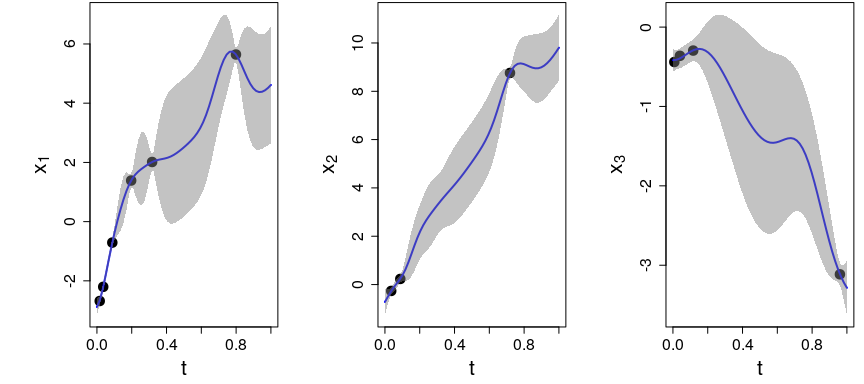Let’s assume that we have additional information for the first two functions by also including their 100th and 150th observations: obsSet[] <- c(5, 10, 23, 50, 80, 100, 150, 200) obsSet[] <- c(10, 23, 100, 150, 180) DataObs$input[] <- Data$input[][obsSet[]] DataObs$input[] <- Data$input[][obsSet[]] DataObs$response[] <- Data$response[][obsSet[], realisation] DataObs$response[] <- Data\$response[][obsSet[], realisation]
predCGP <- mgprPredict(train=res, DataObs=DataObs, DataNew=DataNew)

Now notice how predictions for the third function are affected by the information added to the other functions.

plot(res, DataObs=DataObs, DataNew=DataNew)`